What does it do?

Searches for an approximate value in the first column of a table array and returns a value in the same row from another column (to the right) in the table array.

Formula breakdown:

=VLOOKUP(lookup_value, table_array, col_index_num, [range_lookup])

What it means:

=VLOOKUP(this value, in this list, and get me value in this column, Approximate Match/TRUE/1])

The Vlookup function in Excel is great when you want to find an exact match in your data table but what happens if you want to find an approximate match?

Approximate matches are used when you have an ascending table like Commission Bonus Rates or Income Tax Rates.

IMPORTANT: For the Vlookup Approximate Match to work in Excel, the table_array has to be sorted in ascending order!

So the way that this formula works is that it looks at the first value in the Table_Array that is greater than the Lookup_Value and then goes back one value.

## Want to know how to use VLOOKUP with Approximate Match?

*** Watch our video and step by step guide below with free downloadable Excel workbook to practice ***

Watch on YouTube and give it a thumbs up 👍Have  a look at the following tutorial which explains this formula & don’t forget to download the workbook so you can practice:STEP 1: We need to enter the VLOOKUP function in a blank cell:

## =VLOOKUP(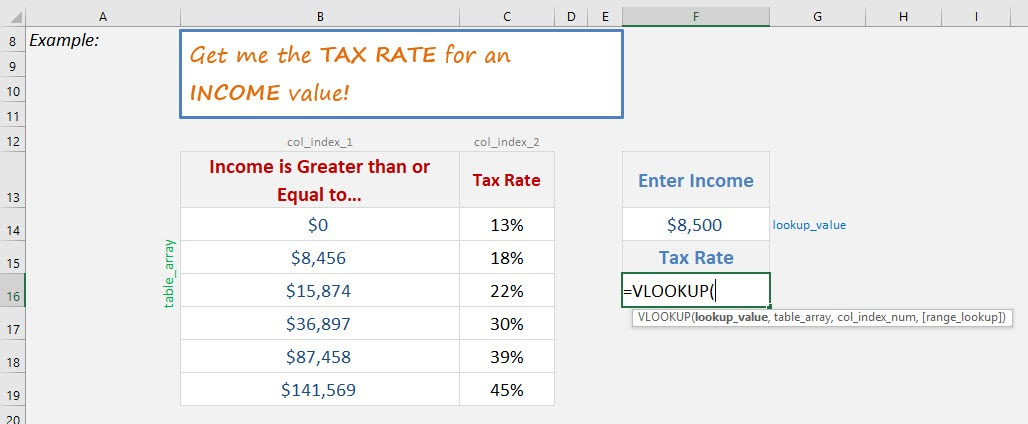STEP 2: The VLOOKUP arguments:

## Lookup_value

What is the value to be looked up?

Select the cell that contains the income as the lookup value.

## =VLOOKUP(F14,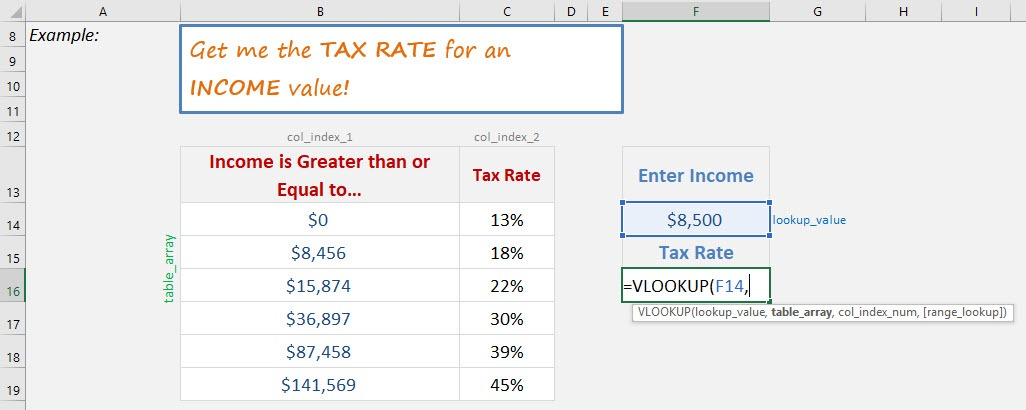## Table_array

Where is the list of data?

Select the tax table, as that is where our formula is going to get the tax rate.

## =VLOOKUP(F14, B14:C19,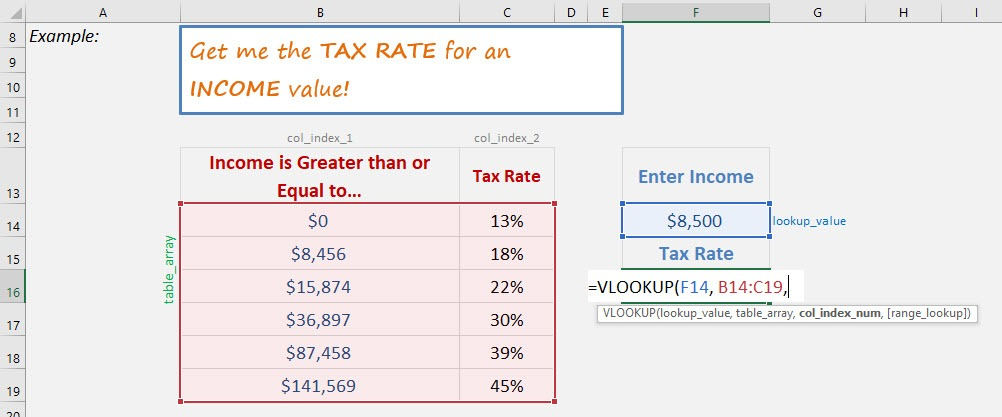## Col_index_num

Which column in the table_array contains the data you want to return?

We want the tax rate which is the second column.

## =VLOOKUP(F14, B14:C19, 2,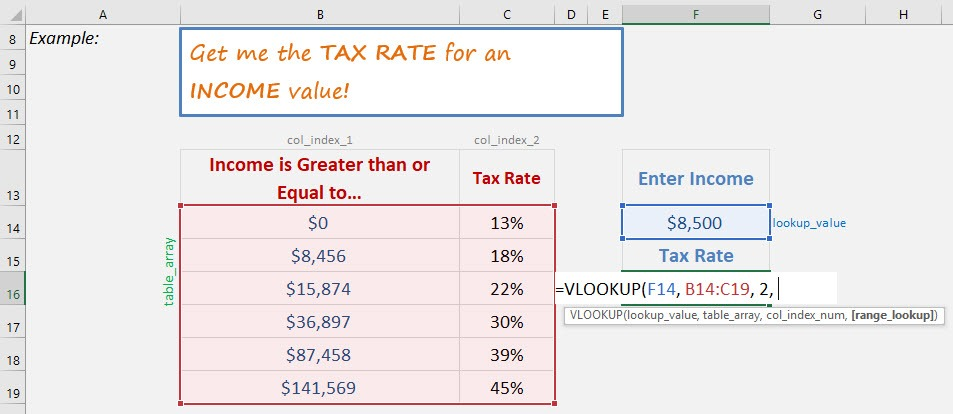## [Range_lookup]

Would it be an approximate match?

Set this to TRUE as we want an approximate match.

## =VLOOKUP(F14, B14:C19, 2, TRUE)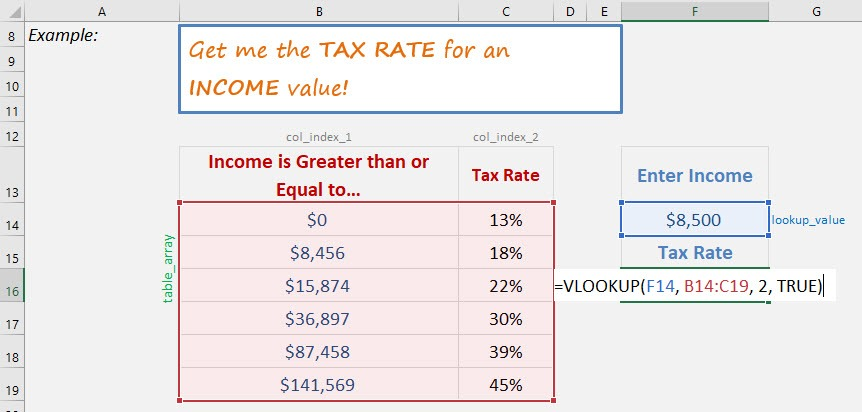You now have your tax rate!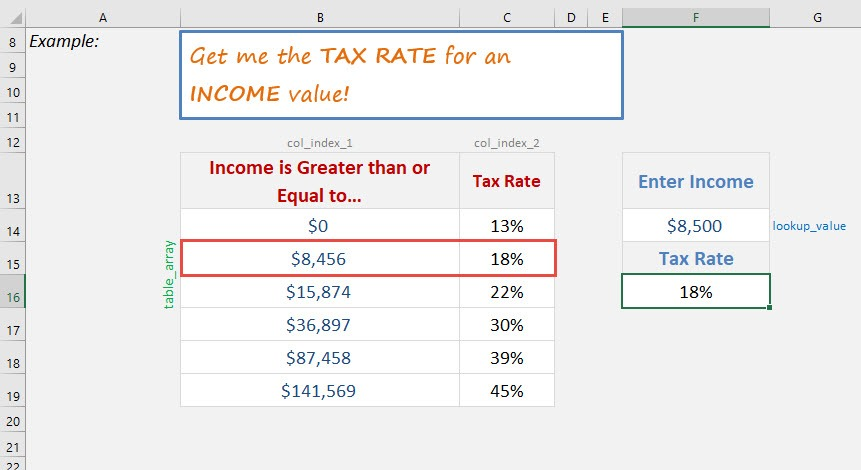How to Do VLOOKUP Approximate Match in Excel### Post Reviews

0
0.0 rating
0 out of 5 stars (based on 0 reviews)
Excellent0%
Very good0%
Average0%
Poor0%
Terrible0%

There are no reviews yet. Be the first one to write one.

### Leave a Review

•Andrew says:

Hi John!

I’m looking for the solution all over the internet without success. I would like to find the approximate match in columns with date and time, but the real approximate match. As You wrote: “So the way that this formula works is that it looks at the first value in the Table_Array that is greater than the Lookup_Value and then goes back one value”, but maybe that greater value is the nearest approximate match. Do You know any solutions for that?
Kind regards,
Andrew

•Bryan says:

Hi Andrew,

Would you mean that instead of going one step lower for the approximate match, you always want the first higher value instead?

Regards,
Bryan

•Osvaldo Labbe says:

Hi John, I’m a Teacher and I`’m looking for material for my Excel classes. So your blog is very interesting for me.
Thank you

•John Michaloudis says:

Hello Osvaldo,

Feel free to share the material on my blog with your students, the more the merrier!

Just make sure to provide a link to the blog or tutorial that you used.

Cheers,
John

•Tom says:

Hi John, I really like the animations you do to explain basic Excel principles. Considering VLOOKUP APPROXIMATE – what about the DOUBLE APPROXIMATE VLOOKUP? It is the best option to go with – feel free to read my 2 cents on it: http://www.analystcave.com/excel-vlookup-how-to-use-vlookup-correctly/

•John Michaloudis says:

Hey Tom,

I LOVE your post which explains VLOOKUP and I am sure that a lot of people will take lots of value from your article.

The double approximate vlookup is the fastest way to get a result and it is something that Charles Williams over at http://www.decisionmodels.com uses all the time for speed.

Cheers,

John